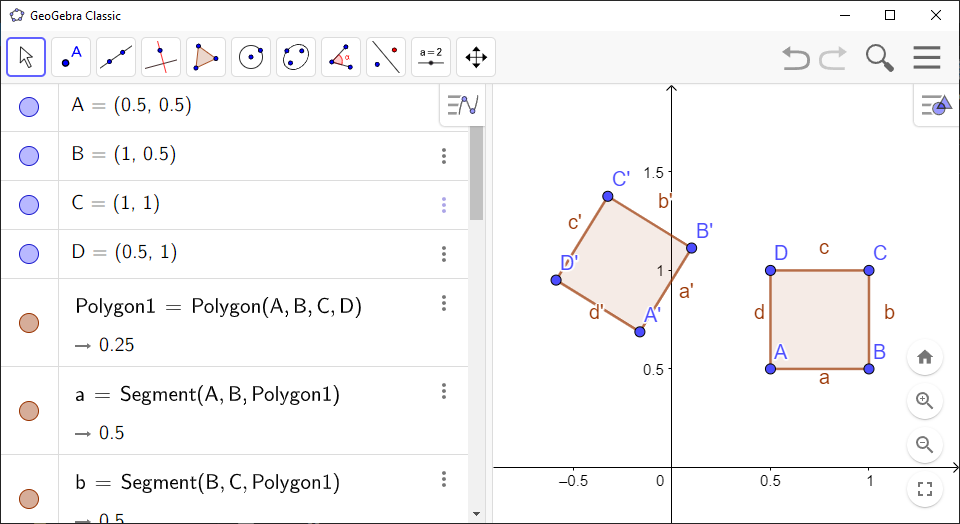Math Topics

# How to Rotate a Figure in GeoGebra

You can use GeoGebra to rotate a figure.

GeoGebra Instruction 1

### RotateaFigure

1.
Open Algebra View and Graphics View underView inMenu.
2.
Draw the figure you want to rotate.
3.
Draw a point. This point will be the point you’ll rotate the figure around in the later steps.
4.
Select the tool Rotate around Point, which you can find by first selecting tool number 9 (Reflect about Line), then the fourth tool in the list.
5.
Click your figure, then the point from Step 3. Your figure has just been rotated!